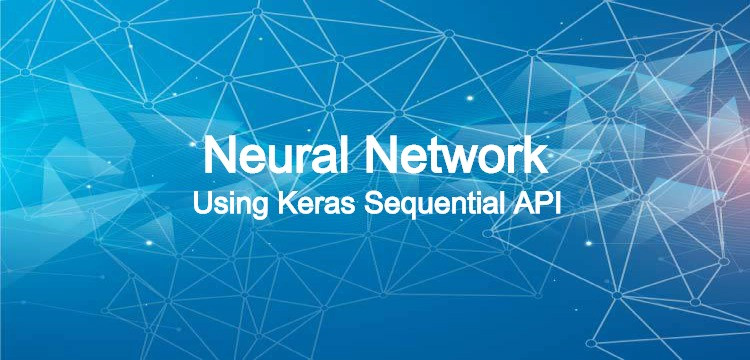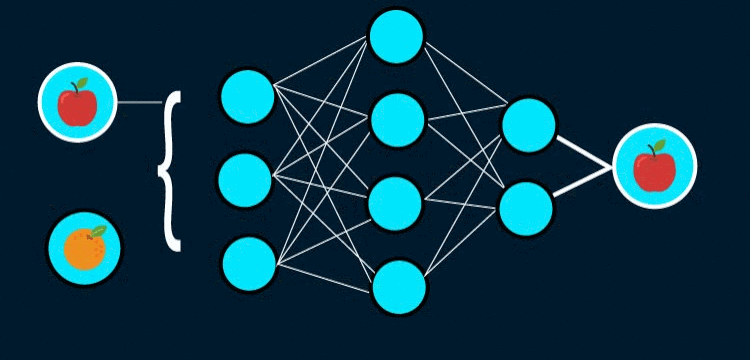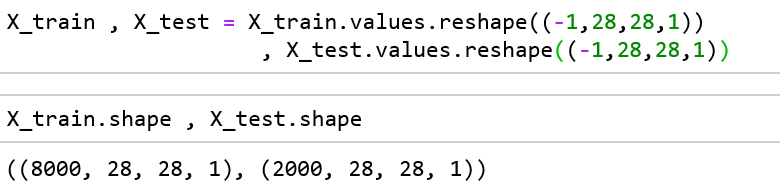Search

# Neural Network Using Keras Sequential API: Overview, Structure, ApplicationsThe main idea behind machine learning is to provide human brain like abilities to our machine, and therefore neural network is like a boon to this ideology. Neural networks are said so because it is inspired by the working of the human brain’s neurons. So, how does the human brain neurons work? And how this structure helped neural networks and deep learning? Let’s discuss this all.

Topics Covered

1. Overview of Neural Network

2. Structure of Neural Network

3. Neural Network Implementation Using Keras Sequential API

4. Application of Neural Network

5. Conclusion

Structure of Neural Network

Let’s see the basic structure of neurons working in the human brain.Working of neuron

Neurons in brains have three most important features -

1. Dendrites: that gives input to the nucleus of the neuron.

2. Nucleus: that processes the information.

3. Axon: that acts as an output layer.Basic neural network structure

Input x1, x2, x3 which contains information in association with weights w1, w2, w3 acts as input layer and is stored in a matrice form known as hidden layers. Further in the structure ∑ shows the activation function which acts as a decision-maker and allows only certain useful information to fire forward further in the network towards the output layer.

Input -> matrix activation -> activation -> output

Here, the activation function decides which feature or information to fire forward towards output in order to minimize error. Generally, the sigmoid function or softmax is seen to be preferred by data scientists and machine learning engineers.

Here is the sigmoid functionOther activation functions that are widely used and accepted are Tanh and softmax.

Now we shall visualizeNeural network architecture

Above is the structure followed by Neural Networks, firstly we have an input layer which includes dataset (either labelled or unlabelled) then there are hidden layers, we can use as many hidden layers as we want as all it does is extraction of informative features from the dataset, we must choose our number of hidden layers wisely as too many features can lead to overfitting which may disturb the accuracy of our model to some extent. Lastly, we have our final layer which is the output layer to give results. For more accuracy, we train our data again and again till then it learns all the features that are required. This information as input is stored as a matrix form which includes information with weight and bias associated with it.

Loss compilation and reducing the loss function is one of the most important work to do in neural networks, we reduce our loss function using a very intuitive algorithm known as gradient descent which finds out the error and minimizes it, in the mathematical statement, it can optimize the convex function.1. Take random 𝚹

2. Update 𝚹 in a direction of decreasing gradient(slope)

3. Update gradients 𝚹 = 𝚹 - ղ*ძf(𝚹) / ძ(𝚹)

Here ղ is learning rate, we have to repeat step 2 until we reach to the local minima.

Like we teach a child when he makes mistakes, our model is also like that child, it makes mistakes and needs someone to teach it whenever it makes mistakes, this is handled by an algorithm known to be Backpropagation.

It works with the help of gradient descent and other functionality. It moves in a backward direction for re-training the network by changing weights and this retraining happens till our model gives us optimum results with the least possible errors. This algorithm is a work of David Rumelhart, when in 1986 he published a famous note on this algorithm, although it introduced a long back in 1970.

Gif visualization of the neural network:Architecture of Neural Network

In the above visualization, two images are provided as an input, our model processes and learn the features of input images, further our model becomes capable of classifying both images on the basis of features it has learned as we can see in our output layer.

Neural Network Implementation Using Keras Sequential API

Enrol now for complete 'Neural Networks with Python' course at just \$2.5 using this link.

Step 1Importing every necessary library, including train_test_split from sklearn and also importing layers like convolutional 2D, Activation, Max pooling, etc.

Step 2:Reading our dataset with the help of the panda's library and visualizing our data. we can analyze the shape of our dataset which contains 1000 rows and 785 columns.

Here is the Dataset

Step 3:In this step we specified x and y, afterwards, we did splitting into training and testing (80% - 20%).

Here np.utils convert a class integer to the binary class matrix for use with categorical cross-entropy.

Step 4:Reshaping our x_train and x_test for use in conv2D. And we can observe the change in the shape of our data.

Step 5:Initializing our model, first addition adds input layer, another layer is hidden layer 1 and next is the output layer. We can observe that we have taken different activation functions such as sigmoid, tanh, and softmax. All these are one of a kind activation function.

Step 6:We can see the output shape at every layer with the number of parameters.

There are none non-trainable parameters which mean every parameter has been analyzed.

Step 7:As commented in our code, we are initializing our weights here.

Step 8:Fitted our training data to our model with 100 epochs and 256 as batch size.

Epochs are the number of times we need to validate our data and batch size which contains all the parameters but are computed simultaneously.

Step 9:Plotting our data, here we can see the slight difference between the loss of our training and testing data, we can also observe the difference between the accuracy of our training and testing data.

Applications of Neural Networks

1. To Solve a Regression Problem - In predicting an accurate continuous value, we can use a simple neural network.

2. For Clustering - If the given dataset is unlabelled or unsupervised, our neural network will form clusters to distinguish classes.

3. Pattern Recognition - There are feedback neural networks which help in tasks like pattern recognition.

4. Dimension Reduction - To understand our data and to extract maximum features out of the data we need to reduce its dimension which can be easily done with the help of artificial neural networks.

5. Machine Translation - We must have used keyboards that translates from one language to another, this is nothing but a machine translation which can be achieved using neural networks.

Conclusion

Neural networks are the foundation of deep learning which helps in performing various tasks, we have learned about the basics of how it works and learned the coding part with the help of Keras functional API.

We would like to thank Analytics Steps for partnering with us in our endeavour to spread Data Science and Machine Learning knowledge. For more blogs keep exploring and keep reading Analytics Steps.